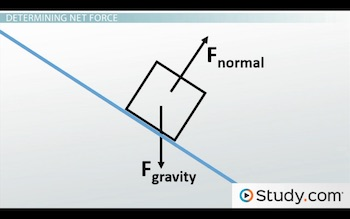# Inclined Planes in Physics: Definition, Facts, and Examples

An error occurred trying to load this video.

Try refreshing the page, or contact customer support.

Coming up next: What is Mechanical Energy? - Definition & Examples

### You're on a roll. Keep up the good work!

Replay
Your next lesson will play in 10 seconds
• 0:01 Tilted Surfaces
• 1:27 Determining Net Force
• 4:26 Friction Affects Net Force
• 6:01 Lesson Summary
Save Save

Want to watch this again later?

Timeline
Autoplay
Autoplay
Speed Speed Audio mode

#### Recommended Lessons and Courses for You

Lesson Transcript
Instructor: Sarah Friedl

Sarah has two Master's, one in Zoology and one in GIS, a Bachelor's in Biology, and has taught college level Physical Science and Biology.

If an object is not horizontal to the ground, it may be on an inclined plane. We need to adjust both the calculations and the free-body diagram when determining the net force on an object on an inclined plane. Learn how in this lesson.

## Tilted Surfaces

When you sit at the top of a slide, you are perpendicular to the ground. But if you scoot far enough forward, you will end up sliding down the slide because it is a tilted surface. So down you go until you reach the bottom, where you (hopefully) end up perpendicular to the ground once again.

The reason you travel down the slide is because of an unbalanced force. This happens for most objects - unbalanced forces cause them to travel down tilted surfaces, which in physics we call inclined planes. Things like balls, toy cars, and dinner plates will all slide down an inclined plane as gravity pulls them back to Earth from their elevated position at the top of the plane. This is because the force of gravity is an object's weight, and it is what keeps us on the ground instead of floating around in the air.

As with objects that are flat on the ground, the normal force, which is a force that acts in the direction perpendicular to a surface, also acts in these situations. Usually, when we're standing on flat ground, the normal force works in the direction opposite to gravity because the ground is horizontal.

But as an object travels down an inclined plane, the normal force is tilted along with the tilt of the plane. So instead of being perpendicular to the ground, it's now perpendicular to the surface of the inclined plane. In fact, the normal force will always be perpendicular to the surface the object is on, no matter the angle of the surface.

## Determining Net Force

This makes things a bit tricky for determining the net force acting on an object because gravity is still directed downward, even when the normal force is not directed upward. We can see how this presents a problem by using a free-body diagram to illustrate the forces.As you can see, the normal force acts perpendicular to the surface of the plane but gravity still acts downward and perpendicular to the horizontal ground.

What we need to do is take that one gravity vector and break it into two components. One component is parallel to the inclined surface and the other is perpendicular to the surface but in the opposite direction of the normal force.

Now the perpendicular gravity vector balances the normal force and the parallel vector accelerates the object down the inclined plane. Because the normal force and the perpendicular force are balanced, the net force on the object is the parallel component of the gravity vector (in the absence of friction, of course).

Since we split the gravity vector into two components, we calculate the magnitude of each force a bit differently than we would for an object on a horizontal surface. On a horizontal surface, the gravitational force is equal to the object's weight, as is the normal force.

But now the parallel force is the mass of the object * g * sin (theta), where g is the acceleration due to gravity (9.8 m/s^2), and theta is the angle of the inclined plane. For the perpendicular vector, the magnitude is the mass of the object * g * cos (theta).

Let's give this a try. Say you have a 500 kg object sliding down an inclined plane tilted upward from the ground at a 45° angle. You can ignore the downward gravitational force because we split it into its components and instead solve for each of these.

So the parallel component is:

500 kg * 9.8 m/s^2 * sin (45)

which gives us 3465 N of force.

The perpendicular component is:

500 kg * 9.8 m/s^2 * cos (45)

which also gives us 3465 N of force.

We know the normal force is also 3465 N because it balances the perpendicular force. Since there is no force acting in the direction opposing the parallel component, the net force is equal to the parallel force, so 3465 N down the incline.

To unlock this lesson you must be a Study.com Member.

### Register to view this lesson

Are you a student or a teacher?

#### See for yourself why 30 million people use Study.com

##### Become a Study.com member and start learning now.
Back
What teachers are saying about Study.com

### Earning College Credit

Did you know… We have over 200 college courses that prepare you to earn credit by exam that is accepted by over 1,500 colleges and universities. You can test out of the first two years of college and save thousands off your degree. Anyone can earn credit-by-exam regardless of age or education level.